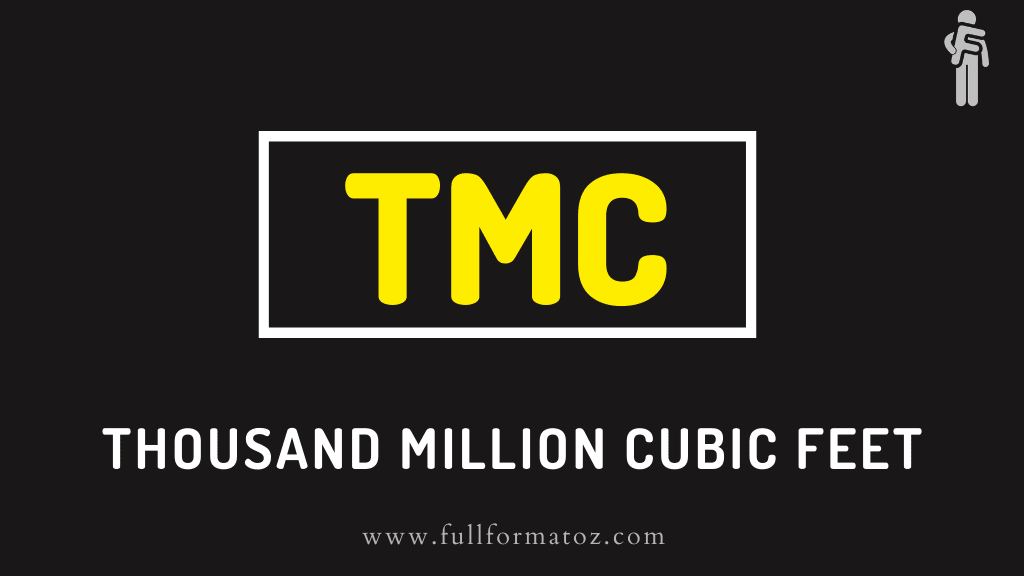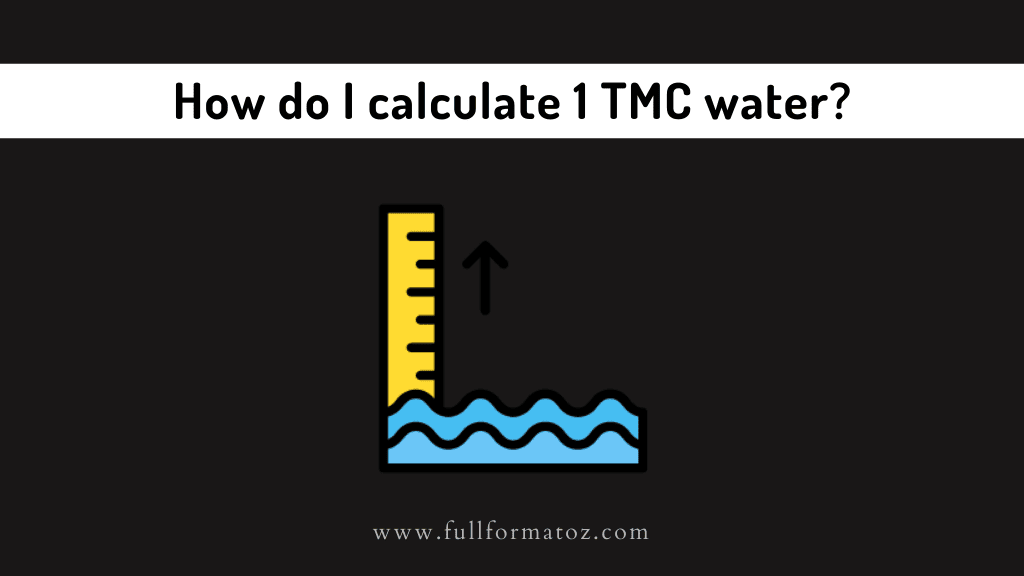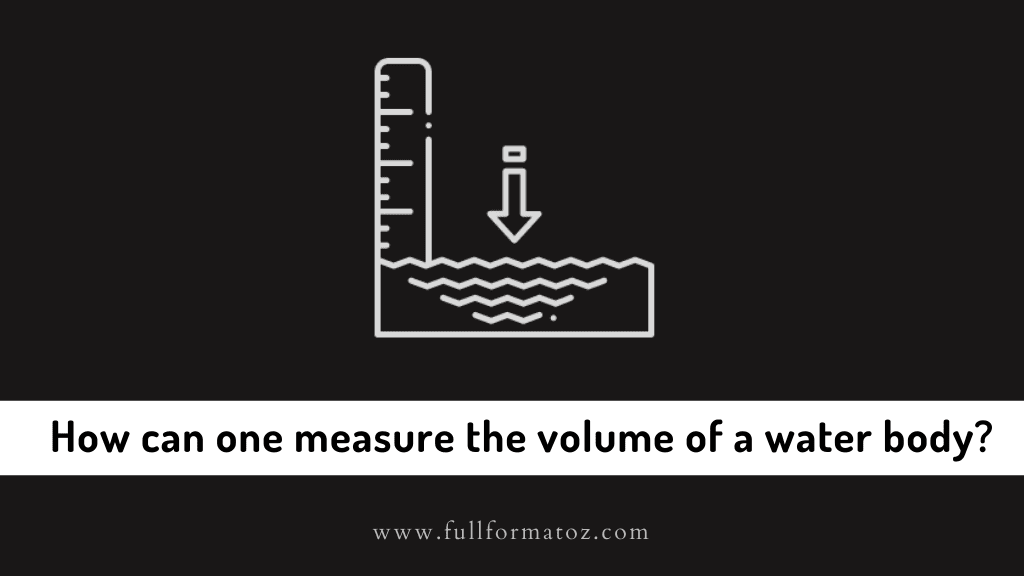# What is the Full Form of TMC in water measurement?## Full Form of TMC in water measurement - Full Form, Meaning, and Definition

The full form of TMC in water measurement is: THOUSAND MILLION CUBIC FEET

### What does TMC mean?

TMC stands for Thousand Million Cubic Feet and is generally used to measure the volume of water in a reservoir. It is also used to measure the inflow and outflow of a dam.

1 TMC = 2831 crore litres = 7478711000 gallon

### How do I calculate 1 TMC water?TMC means a thousand million cubic feet

One Cubic Feet = 1ft x 1ft x 1ft = 0.3048 m x 0.3048 m x 0.3048 m = 0.02831684 m3

We know that 1-meter cube is 1000 liters

Therefore thousand million cubic feet is 0.02831684 x103 x 106m3 or 28.3164 x 109 liters.

1 TCM = 28316846.592 m3

1 TMC = 283.168 Millions litres

### How is water measured in TMC?

Vast water bodies are measured in Thousand Million Cubic Feet or TMC with the help of scientific instruments;

• Clepsydra – Our ancient ancestors used to use this to measure water and to tell time which is now known as the water clock.
• Irrigation – Weir is build surrounding a river or water channel that provides a controlled measure and then ultrasonic transmitters are used to measure the water.
• Global Measurements – Satellites are used to measure huge water bodies like the ocean; boats, and ocean floor surfaces are equipped with electronics and are used to receive and transmit signals.

### How can one measure the volume of a water body?One can measure a small amount of water body by multiplying the length by its width and depth.

For example, the volume of an Olympic-sized swimming pool would be 50 meters into 25 meters to 2 meters. So the pool would hold 2,500,000 liters or 2500 cubic meters of water.

### What is the relation between Cusec and TMC?

A Cusec is a unit meant to measure the flow of liquids, it equals one Cubic foot per second. Hence, Cu(bic) + Sec(ond), a fusion of cubic and seconds. It is expressed as m3/s or m3/s-1.

The amount of water to be discharged through any channel, conduit per sec in cubic feet is known as Cusec. It takes 10^9 seconds to make 1 TMC if a liquid is flowing at a speed of 1 Cusec.

Both of these units are completely different so comparing between them is irrelevant.

1 cubic feet per second = 28.317 liters per second

So, 1 thousand cubic feet = 28,316,846,592 liters